#### WATCH ALL SLIDES

Slide 1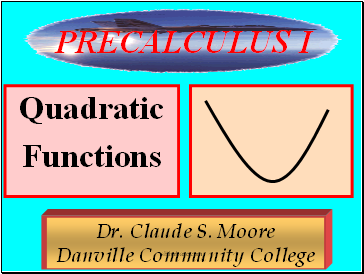Functions

Dr. Claude S. Moore Danville Community College

PRECALCULUS I

Slide 2## Polynomial Function

A polynomial function of degree n is where the a’s are real numbers and the n’s are nonnegative integers and an  0.

Slide 3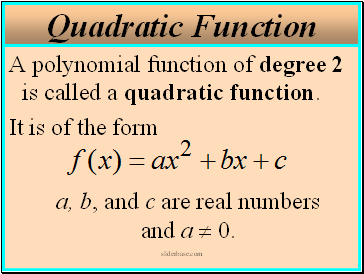A polynomial function of degree 2 is called a quadratic function.

It is of the form a, b, and c are real numbers and a  0.

Slide 4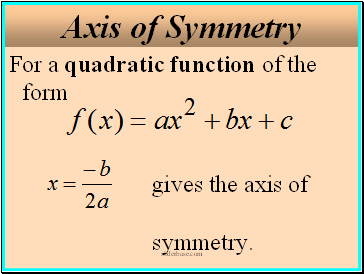## Axis of Symmetry

For a quadratic function of the form gives the axis of symmetry.

Slide 5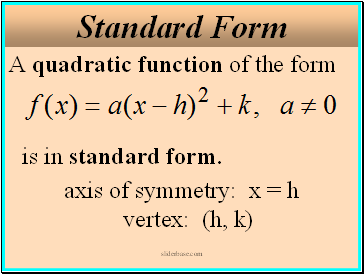## Standard Form

A quadratic function of the form is in standard form.

axis of symmetry: x = h vertex: (h, k)

Slide 6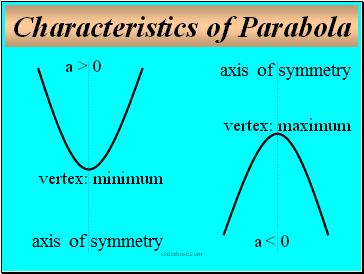## Characteristics of Parabola

a > 0

a < 0

vertex: minimum

vertex: maximum

Slide 7Higher Degree Polynomial Functions

Dr. Claude S. Moore Danville Community College

PRECALCULUS I

Slide 8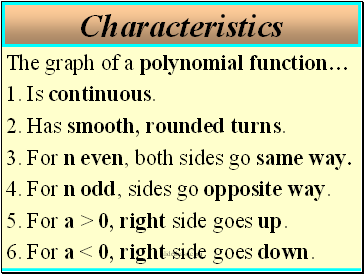## Characteristics

The graph of a polynomial function…

1. Is continuous.

2. Has smooth, rounded turns.

3. For n even, both sides go same way.

4. For n odd, sides go opposite way.

5. For a > 0, right side goes up.

6. For a < 0, right side goes down.

Slide 9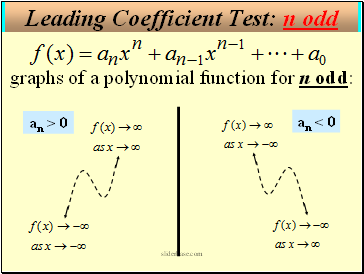.

an < 0

graphs of a polynomial function for n odd:

an > 0

Slide 10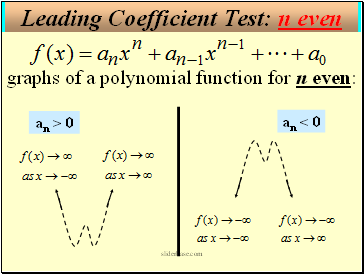.

an < 0

graphs of a polynomial function for n even:

an > 0

Slide 11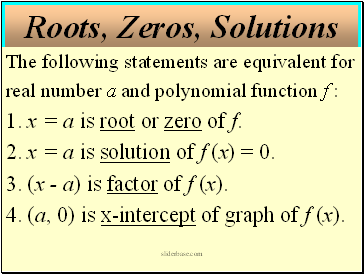## Roots, Zeros, Solutions

The following statements are equivalent for

real number a and polynomial function f :

1. x = a is root or zero of f.

2. x = a is solution of f (x) = 0.

3. (x - a) is factor of f (x).

4. (a, 0) is x-intercept of graph of f (x).

Slide 12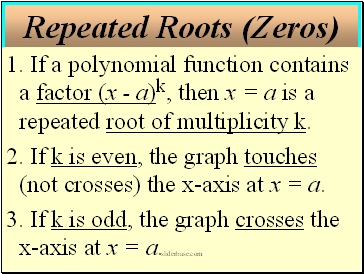1. If a polynomial function contains a factor (x - a)k, then x = a is a repeated root of multiplicity k.

2. If k is even, the graph touches (not crosses) the x-axis at x = a.

Go to page:
1  2  3